# Canvas 中判断点是否在图形上

## 获取坐标

``````canvas.addEventListener('click', (event) => {
const rect = canvas.getBoundingClientRect();
const x = event.clientX - rect.left;
const y = event.clientY - rect.top;

const point = {
x,
y,
}

console.log(point); // 打印点击位置坐标
})

## 几何方法

### 交叉数法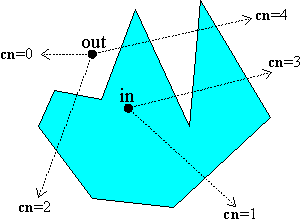``````function getCrossingNumber(point, lines) {
let count = 0;
for (let i = 0; i < lines.length; i += 1) {

// o, d 是多边形某条边的起点和终点
const { o, d } = lines[i];

// 起点和终点位于水平射线的两侧才会有交点
if ((o.y > point.y) ^ (d.y > point.y)) {

// x = (y - y0) / k + x0
const x = (point.y - o.y) * (d.x - o.x) / (d.y - o.y) + o.x;

if (x > point.x) {
count += 1;
}
}
}

return count;
}

Fabric.js 这个库就是使用交叉数法来判断一个点是否在多边形内，具体可以查看代码 github.com/fabricjs/fa…

### 环绕数法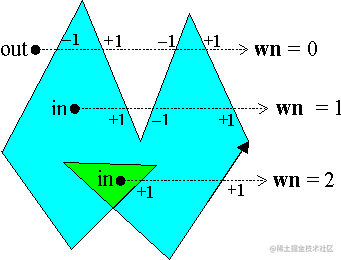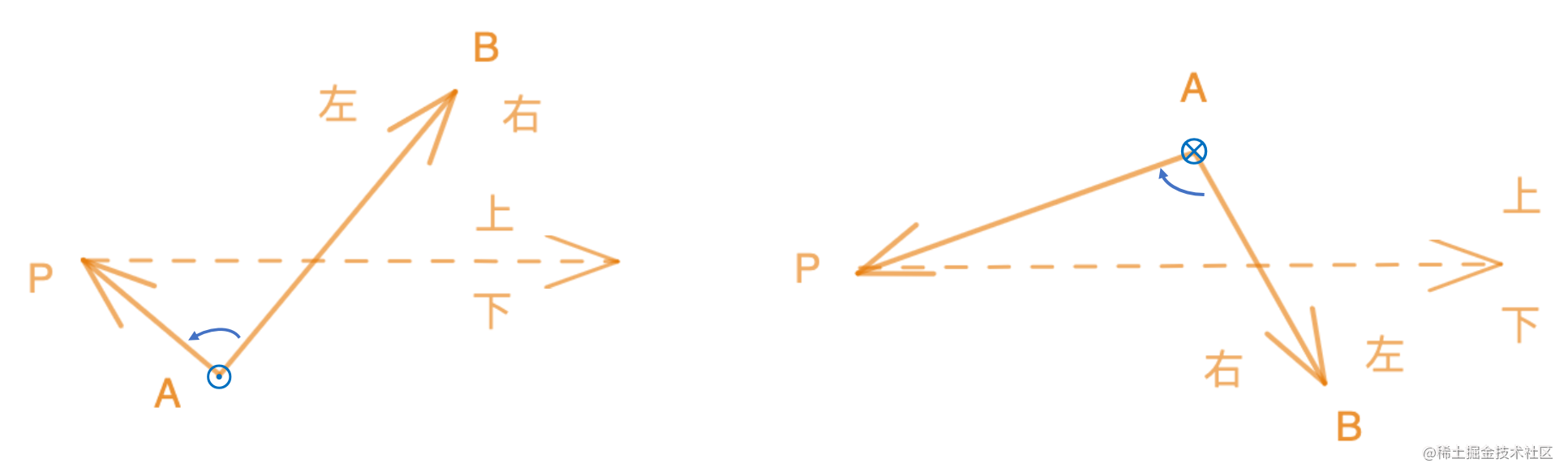``````向量 AB = (x1, y1, 0)

AB × AP = (x1y2 - x2y1)k

• 当 x1y2 - x2y1 > 0 时，P 在 AB 左侧，环绕数 +1
• 当 x1y2 - x2y1 = 0 时，P 在 AB 上，环绕数不变
• 当 x1y2 - x2y1 < 0 时，P 在 AB 右侧，环绕数 -1

## 像素检测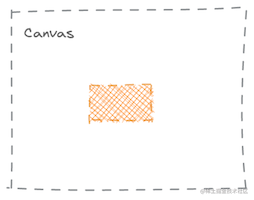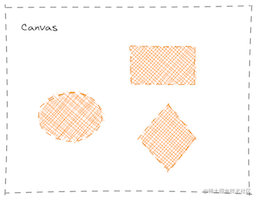### 基于透明度的检测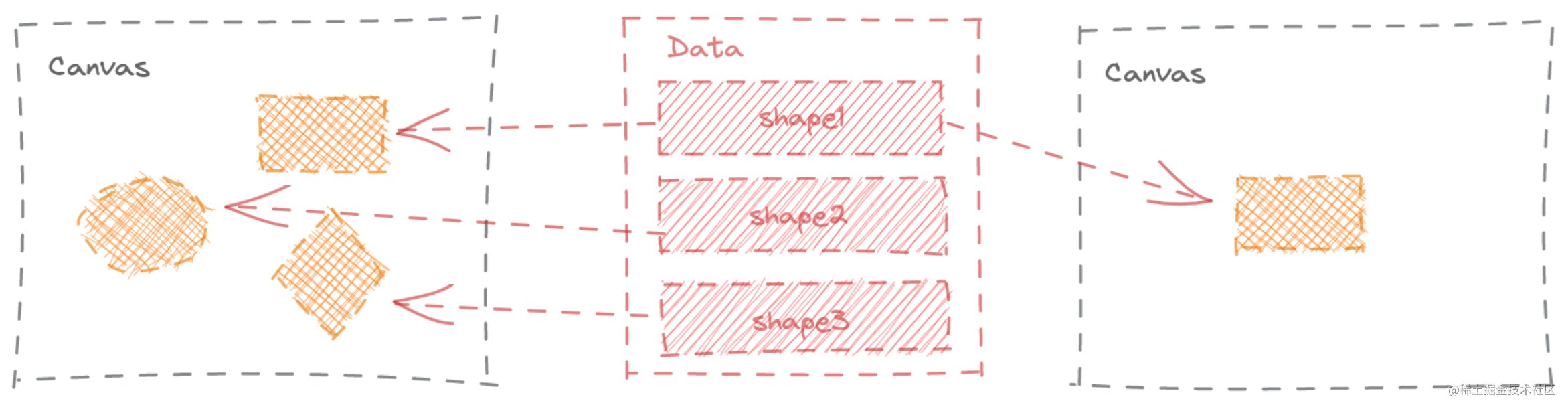Create.js 就是使用的这种透明度对比的方法，来判断某个点是否在某个图形上。这里把 Create.js 的一些代码展示出来，以方便大家的理解。

``````/**
* Tests whether the display object intersects the specified point in local coordinates (ie. draws a pixel with alpha > 0 at
* the specified position). This ignores the alpha, shadow, hitArea, mask, and compositeOperation of the display object.
*
* Please note that shape-to-shape collision is not currently supported by EaselJS.
* @method hitTest
* @param {Number} x The x position to check in the display object's local coordinates.
* @param {Number} y The y position to check in the display object's local coordinates.
* @return {Boolean} A Boolean indicating whether a visible portion of the DisplayObject intersect the specified
* local Point.
*/
p.hitTest = function(x, y) {
// DisplayObject._hitTestContext 是一个新的 Context
var ctx = DisplayObject._hitTestContext;

// 做矩阵变换，将检测的点移到 (0, 0) 的位置
ctx.setTransform(1, 0, 0, 1, -x, -y);

// 在新的 ctx 上绘制出图形
this.draw(ctx, !(this.bitmapCache && !(this.bitmapCache._cacheCanvas instanceof WebGLTexture) ));

// 获取 (0, 0) 位置的透明度，如果透明度大于 1 则被检测的点 (x, y) 在图形上
var hit = ctx.getImageData(0, 0, 1, 1).data > 1;

ctx.setTransform(1, 0, 0, 1, 0, 0);
ctx.clearRect(0, 0, 2, 2);
return hit;
};

### 基于随机颜色的检测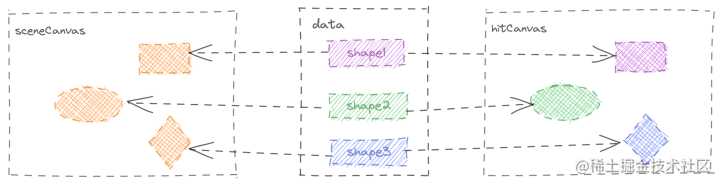Konva.js 采用的是这种方式来判定图形是否点击的。下面的代码是生成随机颜色的代码，点击链接可查看源码， Konva.js 生成随机颜色

``````constructor(config) {
super(config);
// set colorKey
let key;
while (true) {
// 生成随机颜色
key = Util.getRandomColor();
if (key && !(key in shapes)) {
break;
}
}
this.colorKey = key;

// window.Konva.shapes
shapes[key] = this;
}

``````_getIntersection(pos) {
const ratio = this.hitCanvas.pixelRatio;
const p = this.hitCanvas.context.getImageData(Math.round(pos.x * ratio), Math.round(pos.y * ratio), 1, 1).data;
const p3 = p;
// fully opaque pixel
if (p3 === 255) {
const colorKey = Util._rgbToHex(p, p, p);
const shape = shapes[HASH + colorKey];

// 找到对应的图形
if (shape) {
return {
shape: shape,
};
}
return {
antialiased: true,
};
}
else if (p3 > 0) {
// antialiased pixel
return {
antialiased: true,
};
}
// empty pixel
return {};
}

getAllIntersections(pos): get all shapes that intersect a point. Note: because this method must clear a temporary canvas and redraw every shape inside the container, it should only be used for special situations because it performs very poorly. Please use the Konva.Stage#getIntersection method if at all possible because it performs much better